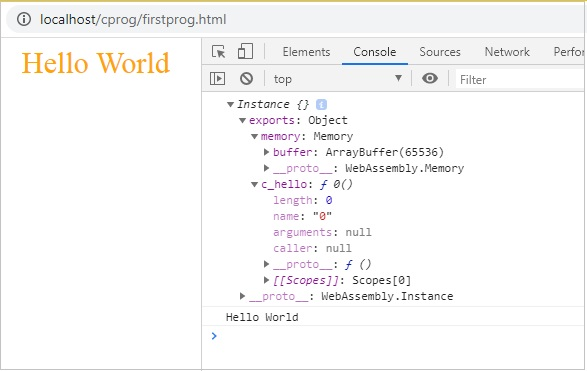# WebAssembly - "Hello World"

In this chapter we are going to write a simple program in C and convert it into .wasm and execute the same in the browser to get the text "Hello World".

Will make use of wasm explorer tool that will convert the C program to .wasm and will make use of the .wasm inside our .html file.

The Wasm explorer tool which is available at https://mbebenita.github.io/WasmExplorer/ looks as follows −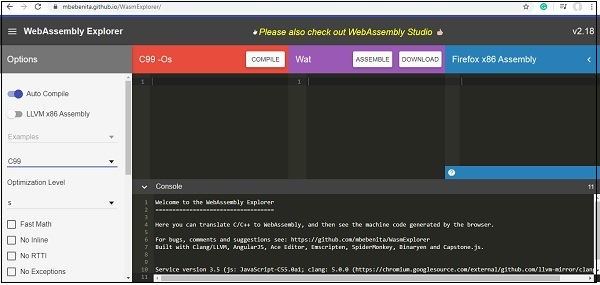The C code that we are going to use is as follows −

```#include <stdio.h>
char *c_hello() {
return "Hello World";
}
```

Update the first block in wasm explorer with the C code as shown below −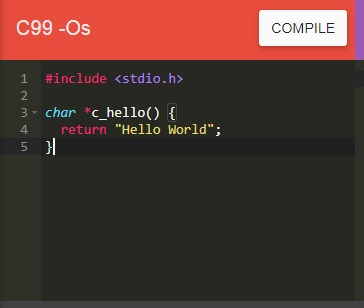Click on COMPILE Button to compile to WASM and WAT and Firefox x86 Web Assembly as shown below −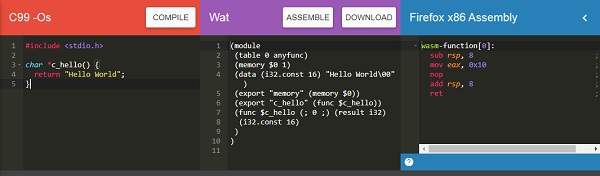Use the DOWNLOAD to get the .wasm file and save it as firstprog.wasm.

Create a .html file called firstprog.html as shown below −

```<!doctype html>
<html>
<meta charset="utf-8">
<title>WebAssembly Hello World</title>
<body>
<div id="textcontent"></div>
<script type="text/javascript">
</script>
</body>
</html>
```

Let us now use firstprog.wasm to read the helloworld from the C function c_hello().

### Step 1

Use fetch() api to read the firstprog.wasm code.

### Step 2

The .wasm code has to be converted into arraybuffer by using ArrayBuffer. The ArrayBuffer object will return you a fixed length binary data buffer.

The code so far will be as follows −

```<script type="text/javascript">
fetch("firstprog.wasm") .then(bytes => bytes.arrayBuffer())
</script>
```

### Step 3

The bytes from ArrayBuffer have to be compiled into a module by using WebAssembly.compile(buffer) function.

The code will look like below −

```<script type="text/javascript">
fetch("firstprog.wasm")
.then(bytes => bytes.arrayBuffer())
.then(mod => WebAssembly.compile(mod))
</script>
```

### Step 4

To get the module we have to call the webassembly.instance constructor as shown below −

```<script type="text/javascript">
fetch("firstprog.wasm")
.then(bytes => bytes.arrayBuffer())
.then(mod => WebAssembly.compile(mod))
.then(module => {return new WebAssembly.Instance(module) })
</script>
```

### Step 5

Let us now console the instance to see the details in the browser.

```<script type="text/javascript">
fetch("firstprog.wasm") .then(bytes => bytes.arrayBuffer())
.then(mod => WebAssembly.compile(mod)) .then(module => {
return new WebAssembly.Instance(module)
})
.then(instance => {
console.log(instance);
});
</script>
```

The console.log details are shown below −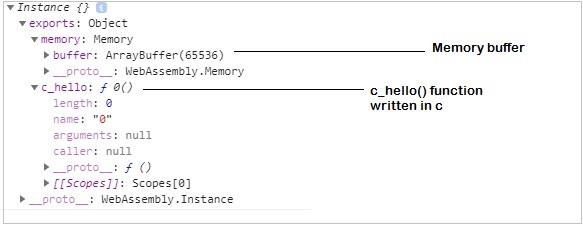To get the string “Hello World” from the function c_hello(), we need to add some code in javascript.

First, get the memory buffer details as shown below −

```let buffer = instance.exports.memory.buffer;;
```

The buffer value has to be converted to a typed array so that we can read the values from it. The buffer has the string Hello World in it.

To convert to typed call the constructor Uint8Array as shown below −

```let buffer = new Uint8Array(instance.exports.memory.buffer);
```

Now, we can read the value from the buffer in a for - loop.

Let us now get the start point to read the buffer, by calling the function we wrote as shown below −

```let test = instance.exports.c_hello();
```

Now, the test variable has the start point to read our string. WebAssembly does not have anything for string values, everything is stored as integers.

So when, we read the value from the buffer, they will be an integer value and we need to convert it into a string using fromCharCode() in javascript.

The code is as follows −

```let mytext = "";
for (let i=test; buffer[i]; i++){
mytext += String.fromCharCode(buffer[i]);
}
```

Now, when you console mytext you should see the string “Hello World”.

## Example

The complete code is as follows −

```<!doctype html>
<html>
<meta charset="utf-8">
<style>
div {
font-size : 30px; text-align : center; color:orange;
}
</style>
<body>
<div id="textcontent"></div>
<script>
fetch("firstprog.wasm")
.then(bytes => bytes.arrayBuffer())
.then(mod => WebAssembly.compile(mod))
.then(module => {return new WebAssembly.Instance(module)})
.then(instance => {
console.log(instance);
let buffer = new Uint8Array(instance.exports.memory.buffer);
let test = instance.exports.c_hello();
let mytext = "";
for (let i=test; buffer[i]; i++) {
mytext += String.fromCharCode(buffer[i]);
}
console.log(mytext); document.getElementById("textcontent").innerHTML = mytext;
});
</script>
</body>
</html>
```

We have added a div and the content is added to the div, so the string is displayed on the browser.

## Output

The output is mentioned below −